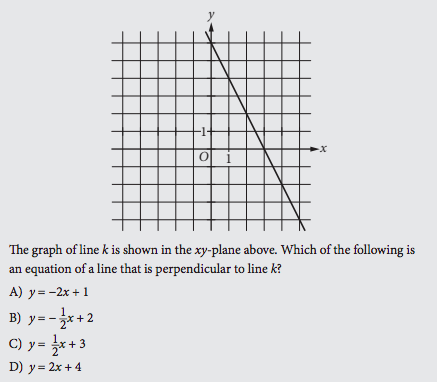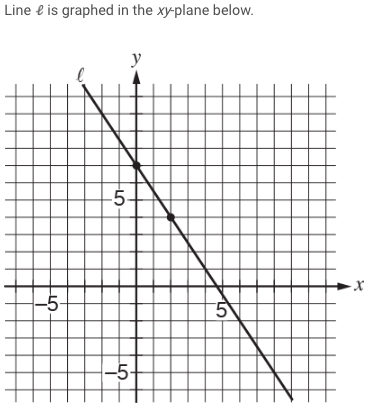### What are your chances of acceptance?Duke UniversityUCLA
Unweighted GPA: 3.7
1.0
4.0
SAT: 720 math
200
800
| 800 verbal
200
800

#### Extracurriculars

Low accuracy (4 of 18 factors)Laura Berlinsky-Schine
3

# SAT Math Prep: Lines and Slopes

## Is your SAT score enough to get you into your dream school?

Our free chancing engine takes into consideration your SAT score, in addition to other profile factors, such as GPA and extracurriculars. Create a free account to discover your chances at hundreds of different schools.

The SAT Math section can be challenging for many students. Fortunately, knowing what types of problems you’ll need to tackle and equations you’ll need to memorize is half the battle.

Lines and slopes are important concepts for the Heart of Algebra section of your math SAT. How can you prepare for this type of problem? Keep reading to learn all about lines and slopes, equations you should know, and examples (with explanations) to help you practice.

## What are Lines and Slopes?

A line is a one-dimensional figure that is completely straight with no curvature and no thickness. Made up of connected dots, it extends infinitely in both directions.

A slope, otherwise known as a gradient, is the measure of the steepness or slant of a line. It is the ratio of the rise (the change in distance vertically over the $$y$$-axis) to the run (the change in distance along the $$x$$-axis).

## What Line and Slope Properties Should You Know?

Before you attempt to tackle problems or memorize equations involving lines and slopes on your SAT, it’s important to understand some fundamental properties of these concepts. The basics include:

• Lines may increase or decrease and be horizontal or vertical.

• A line that increases in height from left to right has a positive slope. A line that decreases in height (gets lower) from left to right has a negative slope.

• A horizontal line has a slope of zero.

• A vertical line has an undefined slope.

• Parallel lines have the same slope and will never intersect.

• Perpendicular lines meet at right angles and have slopes that are negative reciprocals. This means that the product of slopes of perpendicular lines is $$-1$$.

## Lines and Slopes Formulas to Know

Formulas are provided on the SAT, but it will save you time to memorize important ones beforehand. That way, you can tackle the problems without having to flip back to check the formulas.

The equation of a line (also known as slope-intercept form):

$$y=mx+b$$

$$m$$ is the slope and $$b$$ is the $$y$$-intercept. It’s a good idea to rewrite any line equation into the form above to simplify solving any questions.

To find the slope:

$$\frac{y_2-y_1}{x_2-x_1}$$

This represents the change in $$y$$-values divided by the change in $$x$$-values. $$(x_1, y_1)$$ is a coordinate pair from the line, and $$(x_2, y_2)$$ is another coordinate pair from the same line.

As part of our free guidance platform, our Admissions Assessment tells you what schools you need to improve your SAT score for and by how much. Sign up to get started today.

## Examples of Lines and Slopes SAT Problems

Example #1In the above problem, line $$k$$ passes through points $$(0,6)$$ and $$(3,0)$$.

Remember the formula for finding the slope of a line:

$$\frac{y_2-y_1}{x_2-x_1}$$

So in this case, the equation is

$$\frac{6-0}{0-3}$$

The result is $$-2$$.

Remember that the slopes of perpendicular lines have a product of $$-1$$, or must be negative reciprocals of each other. Therefore, the line perpendicular to line $$k$$ must have a slope of $$\frac{1}{2}$$.

In slope-intercept form, the coefficient of x is the slope of the line. So the correct answer is C, the equation with a slope of $$\frac{1}{2}: y=\frac{1}{2}x + 3$$.

Example #2If line ℓ is translated up $$5$$ units and right $$7$$ units, then what is the slope of the new line?

A) $$\frac{2}{5}$$

B) $$\frac{−3}{2}$$

C) $$\frac{−8}{9}$$

D) $$\frac{−11}{14}$$

$$\frac{y_2-y_1}{x_2-x_1}$$

The key to answering this problem quickly and correctly is to remember that translating the line (moving the line somewhere else) will not change the slope of the line. Moving the line up $$5$$ units and over $$7$$ only changes the coordinates of line ℓ, but not the slope.

So, using the original points on the graph as indicators, $$(2,4)$$ and $$(0,7)$$, the slope of line ℓ would be:

$$\frac{4-7}{2-0}$$

In this case, the slope is $$\frac{-3}{2}$$, so the correct answer is B) $$\frac{-3}{2}$$.

## Wrapping it Up

For more practice questions to study up for the SAT, check out the 15 Hardest SAT Math Questions.

Looking for help acing your SAT? Download our free guide with our top 8 tips for mastering the SAT.Short Bio
Laura Berlinsky-Schine is a freelance writer and editor based in Brooklyn with her demigod/lab mix Hercules. She specializes in education, technology and career development. She also writes satire and humor, which has appeared in Slackjaw, Points in Case, Little Old Lady Comedy, Jane Austen’s Wastebasket, and Funny-ish. View her work and get in touch at: www.lauraberlinskyschine.com.